# Optical Instruments Questions and Answers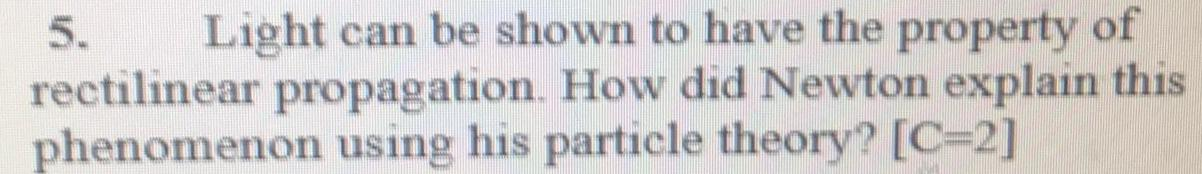Physics
Optical Instruments
Light can be shown to have the property of rectilinear propagation. How did Newton explain this phenomenon using his particle theory? [C=2]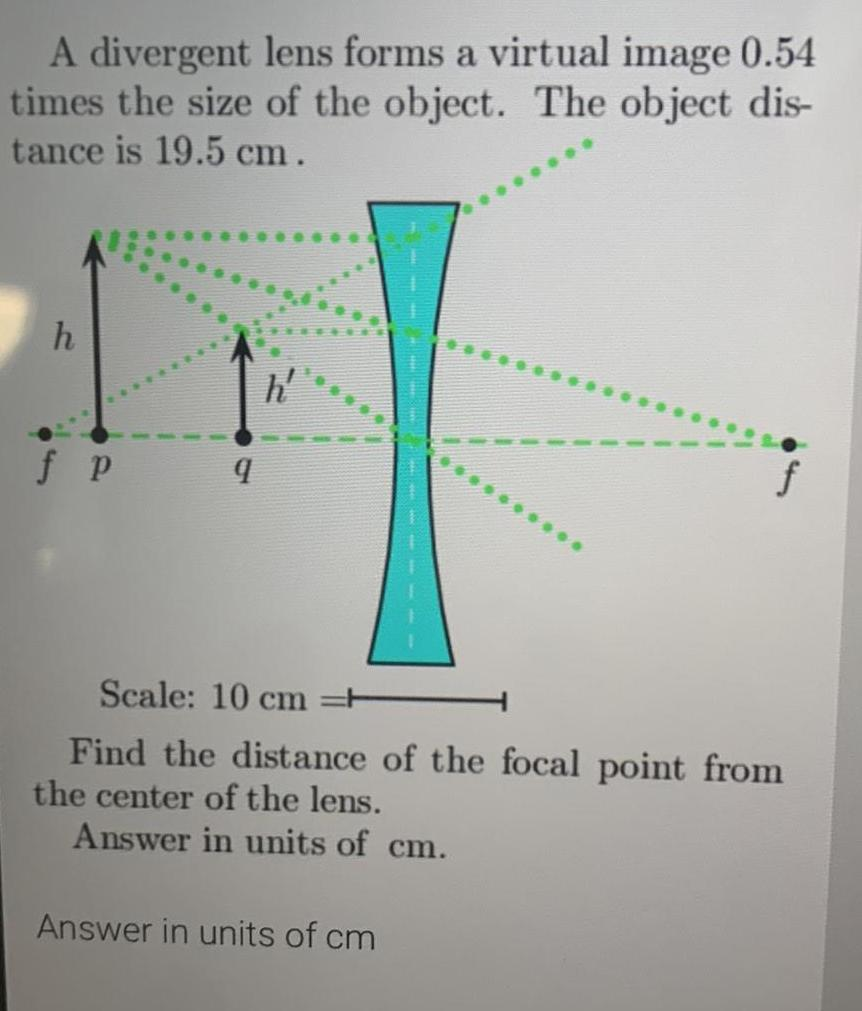Physics
Optical Instruments
A divergent lens forms a virtual image 0.54 times the size of the object. The object dis- tance is 19.5 cm . Scale: 10 cm Find the distance of the focal point from the center of the lens. Answer in units of cm.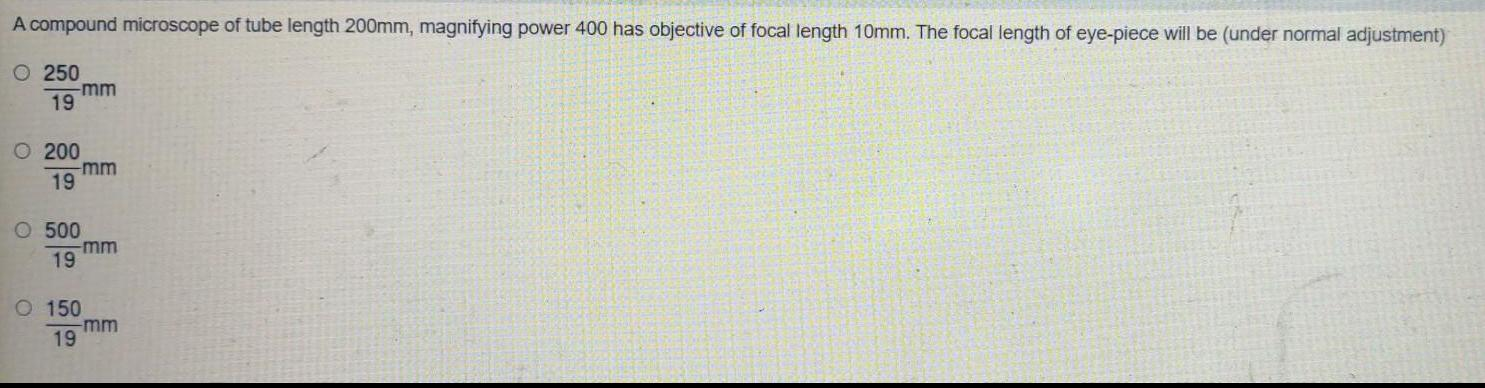Physics
Optical Instruments
A compound microscope of tube length 200mm, magnifying power 400 has objective of focal length 10mm. The focal length of eye-piece will be (under normal adjustment) 250/19 mm 200/19 500/19 150/19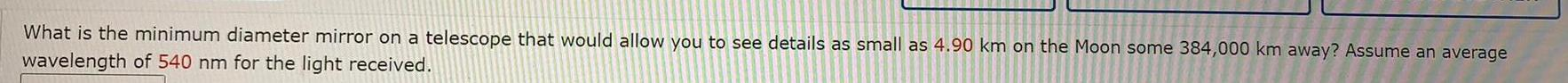Physics
Optical Instruments
What is the minimum diameter mirror on a telescope that would allow you to see details as small as 4.90 km on the Moon some 384,000 km away? Assume an average wavelength of 540 nm for the light received.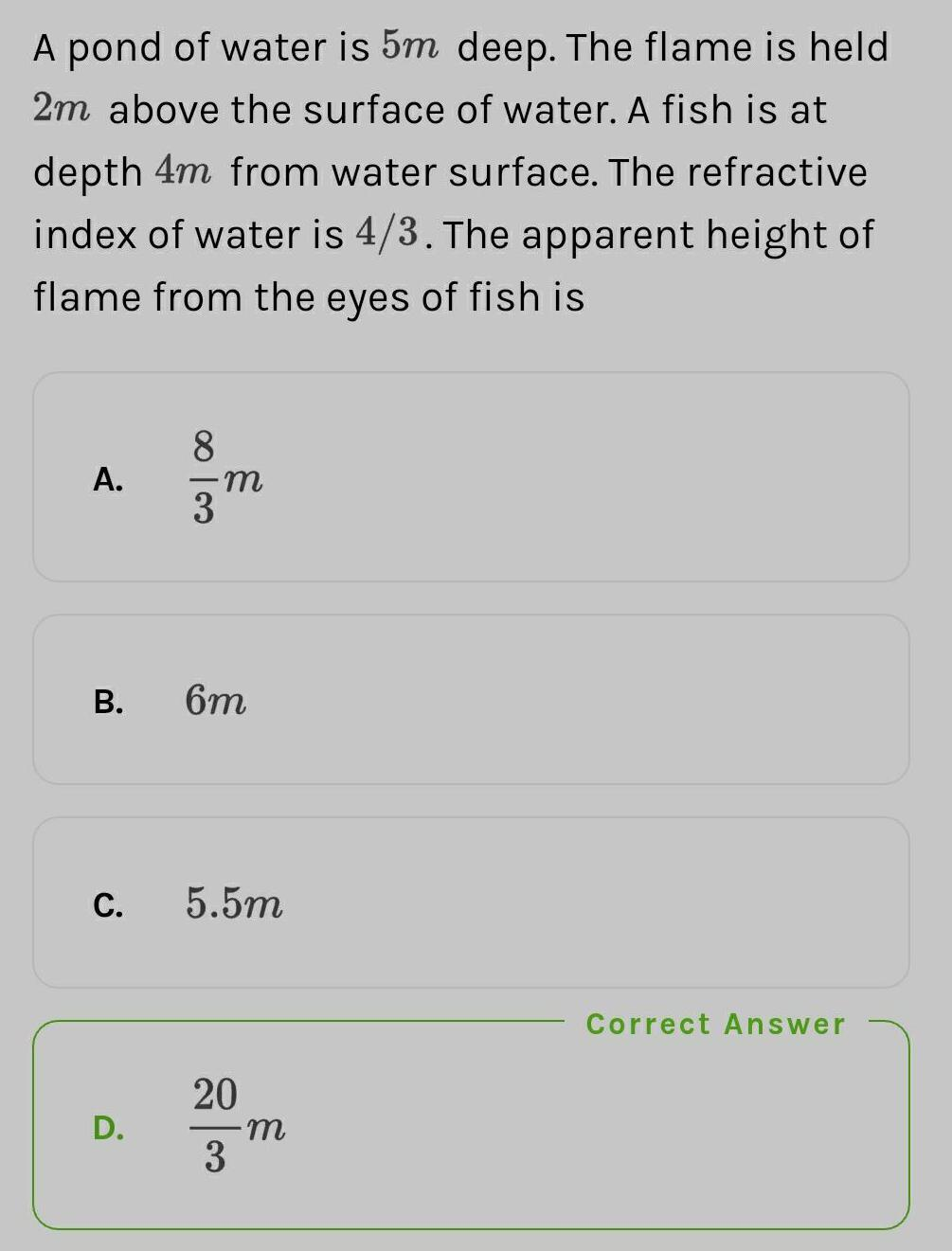Physics
Optical Instruments
A pond of water is 5m deep. The flame is held 2m above the surface of water. A fish is at depth 4m from water surface. The refractive index of water is 4/3. The apparent height of flame from the eyes of fish is Correct Answer A. 8/3m B. 6m C. 5.5m D. 20/3m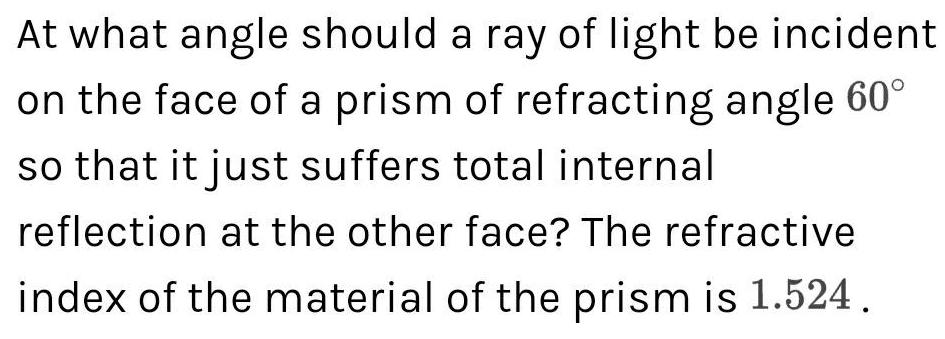Physics
Optical Instruments
At what angle should a ray of light be incident on the face of a prism of refracting angle 60° so that it just suffers total internal reflection at the other face? The refractive index of the material of the prism is 1.524.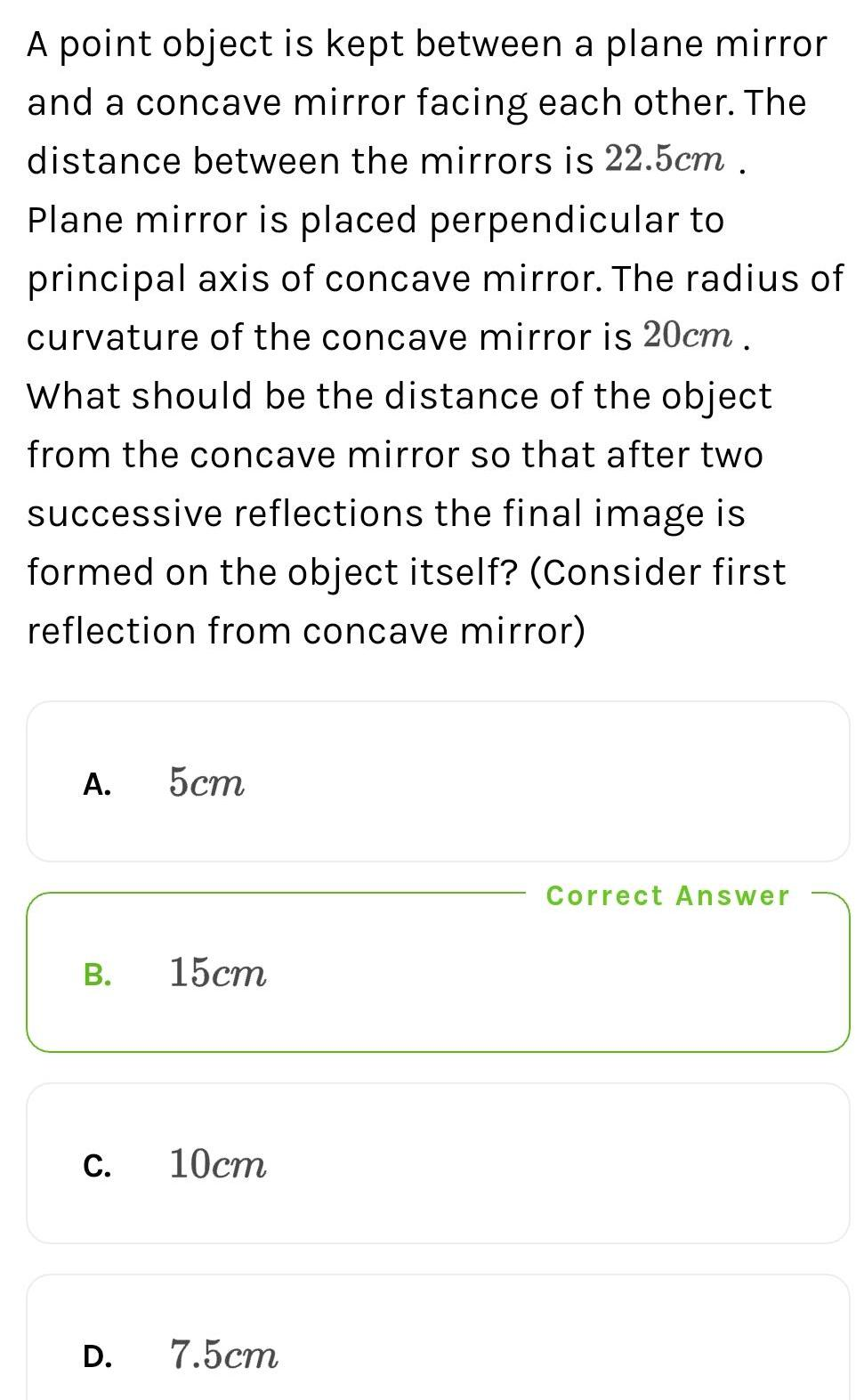Physics
Optical Instruments
A point object is kept between a plane mirror and a concave mirror facing each other. The distance between the mirrors is 22.5cm . Plane mirror is placed perpendicular to principal axis of concave mirror. The radius of curvature of the concave mirror is 20cm. What should be the distance of the object from the concave mirror so that after two successive reflections the final image is formed on the object itself? (Consider first reflection from concave mirror) A. 5cm B. 15cm C. 10cm D.7.5cm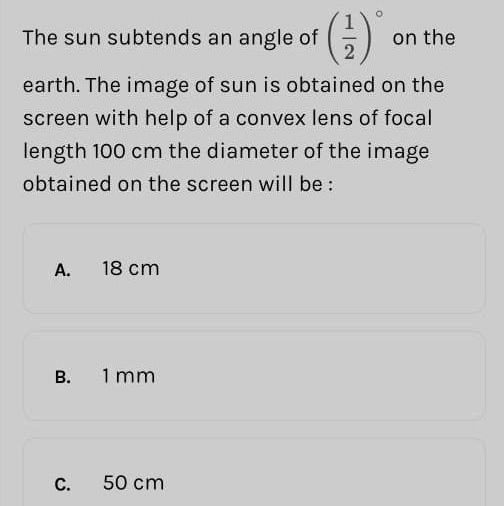Physics
Optical Instruments
The sun subtends an angle of 1.2 on the earth. The image of sun is obtained on the screen with help of a convex lens of focal length 100 cm the diameter of the image obtained on the screen will be: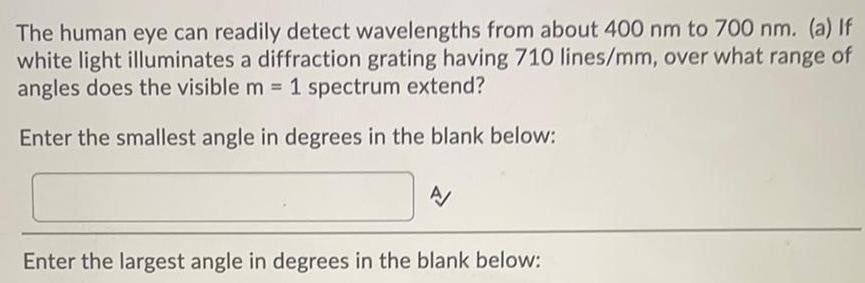Physics
Optical Instruments
The human eye can readily detect wavelengths from about 400 nm to 700 nm. (a) If white light illuminates a diffraction grating having 710 lines/mm, over what range of angles does the visible m = 1 spectrum extend? Enter the smallest angle in degrees in the blank below: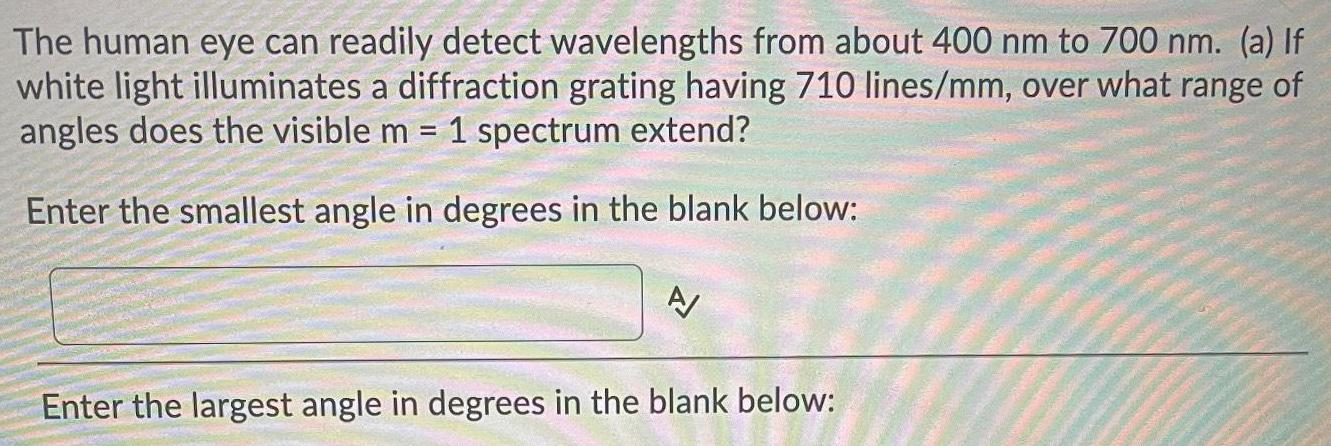Physics
Optical Instruments
The human eye can readily detect wavelengths from about 400 nm to 700 nm. (a) If white light illuminates a diffraction grating having 710 lines/mm, over what range of angles does the visible m = 1 spectrum extend? Enter the smallest angle in degrees in the blank below: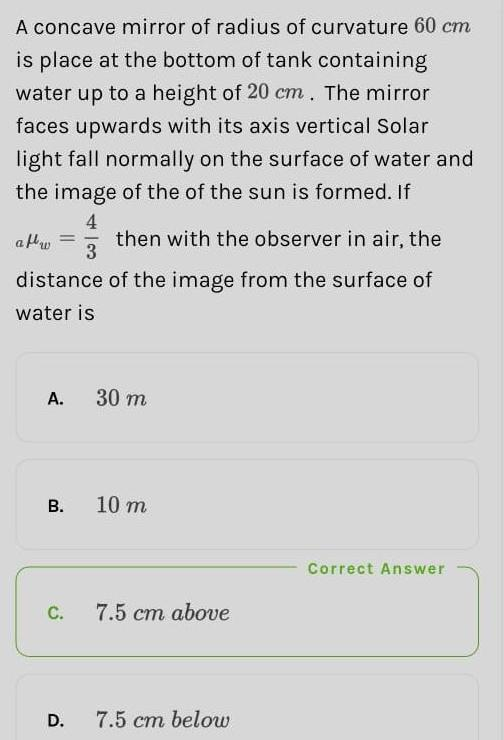Physics
Optical Instruments
A concave mirror of radius of curvature 60 cm is place at the bottom of tank containing water up to a height of 20 cm. The mirror faces upwards with its axis vertical Solar light fall normally on the surface of water and the image of the of the sun is formed. If aµw= 4/3 then with the observer in air, the distance of the image from the surface of water is 30 m 10 m 7.5 cm above 7.5 cm below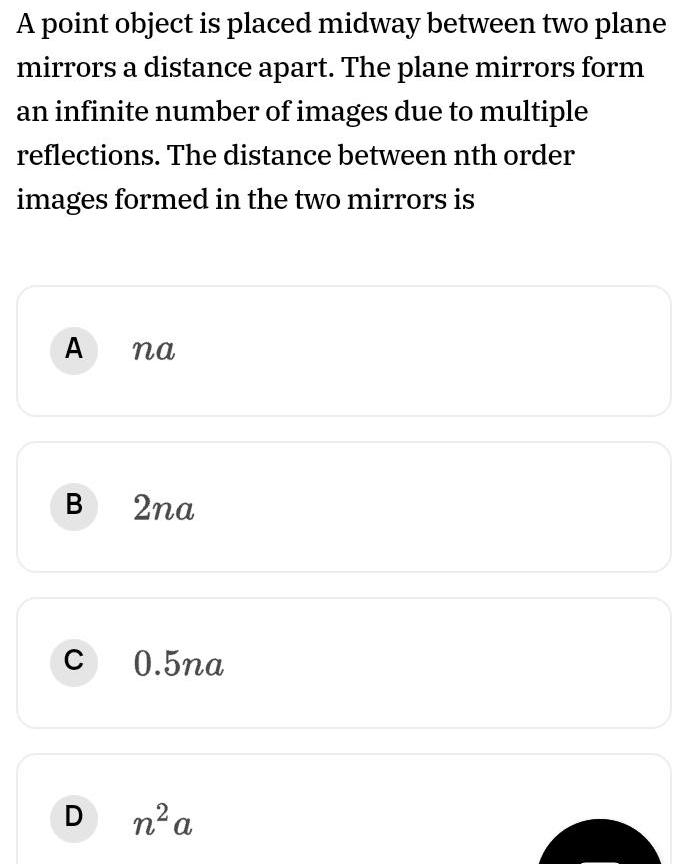Physics
Optical Instruments
A point object is placed midway between two plane mirrors a distance apart. The plane mirrors form an infinite number of images due to multiple reflections. The distance between nth order images formed in the two mirrors is A. na B. 2na C. 0.5na D. n² a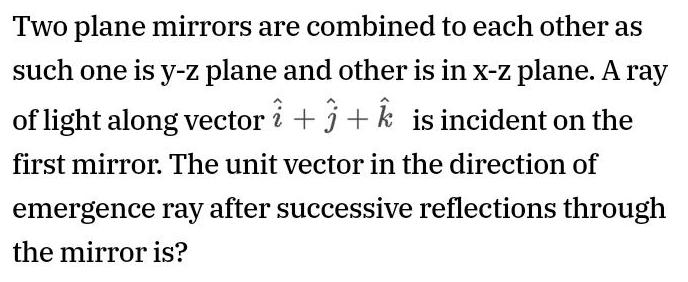Physics
Optical Instruments
Two plane mirrors are combined to each other as such one is y-z plane and other is in x-z plane. A ray of light along vector i+j+k is incident on the first mirror. The unit vector in the direction of emergence ray after successive reflections through the mirror is?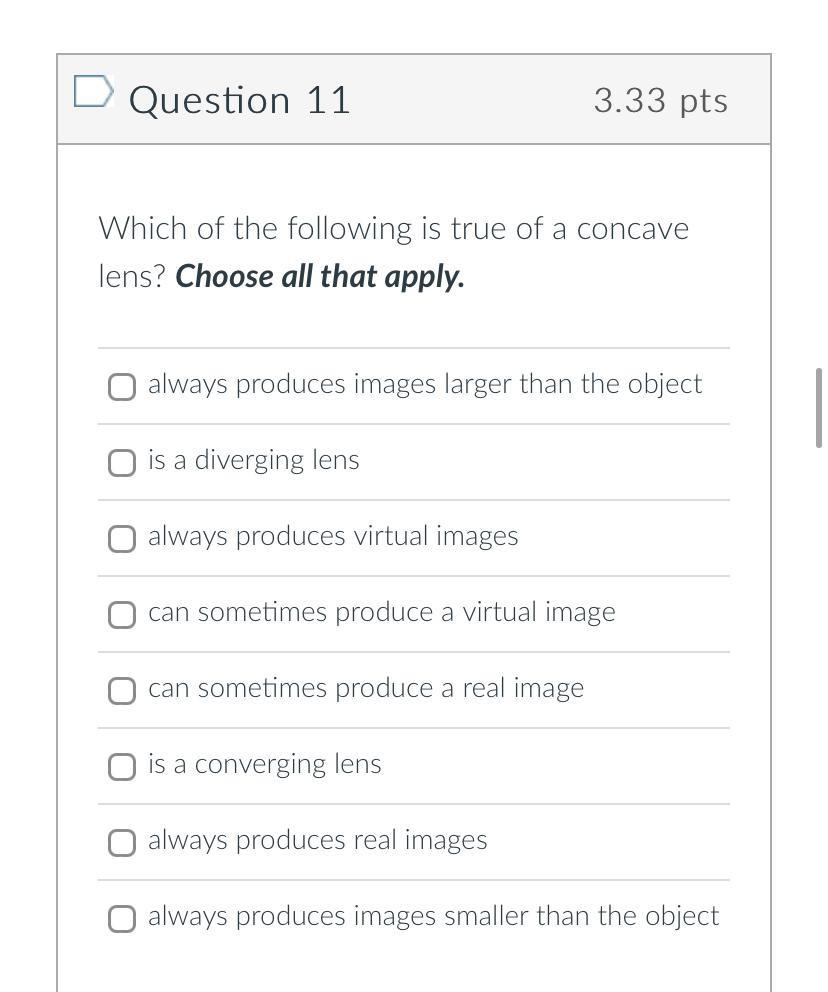Physics
Optical Instruments
Which of the following is true of a concave lens? Choose all that apply. always produces images larger than the object is a diverging lens always produces virtual images can sometimes produce a virtual image can sometimes produce a real image is a converging lens always produces real images always produces images smaller than the object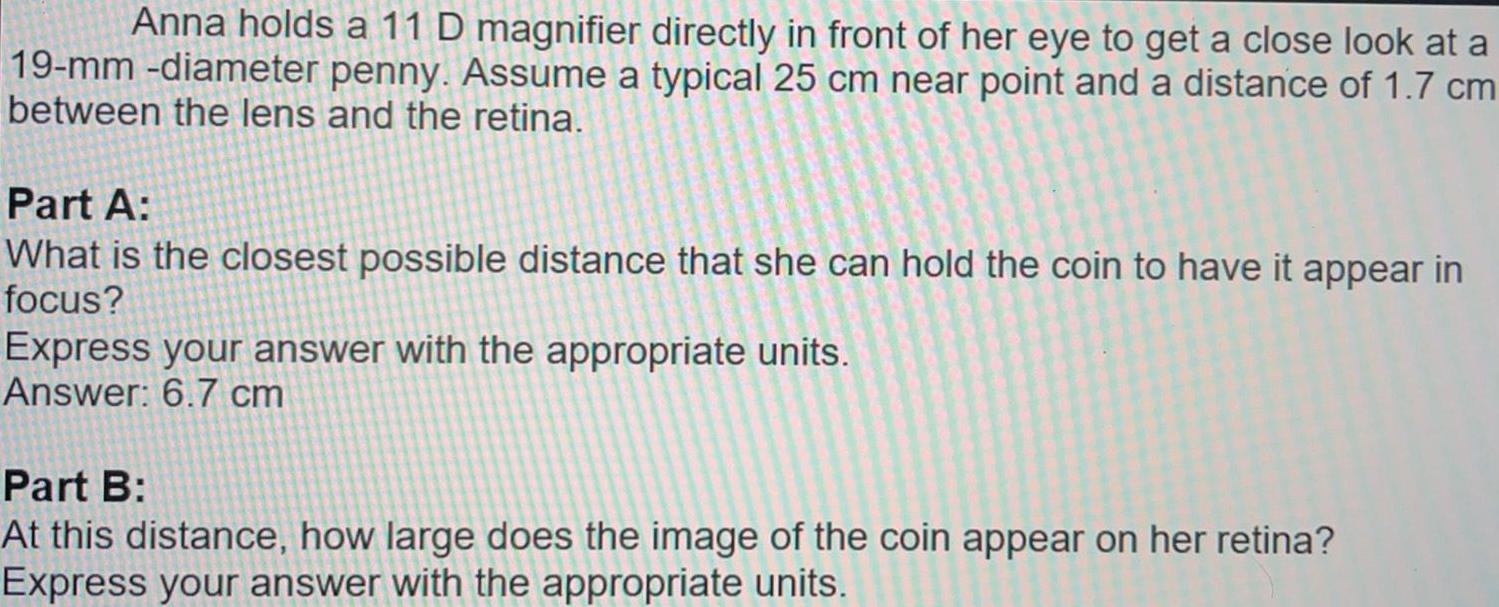Physics
Optical Instruments
Anna holds a 11 D magnifier directly in front of her eye to get a close look at a 19-mm -diameter penny. Assume a typical 25 cm near point and a distance of 1.7 cm between the lens and the retina. Part A: What is the closest possible distance that she can hold the coin to have it appear in focus? Express your answer with the appropriate units. Answer: 6.7 cm Part B: At this distance, how large does the image of the coin appear on her retina? Express your answer with the appropriate units.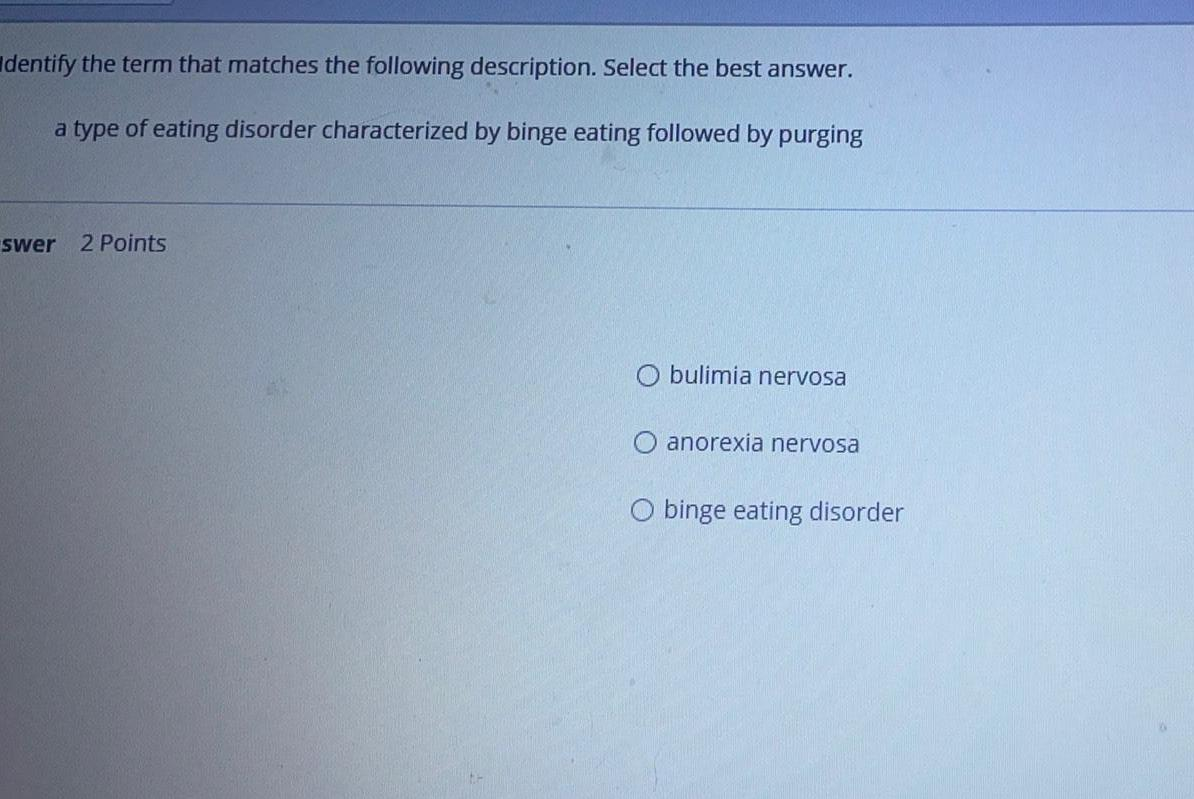Physics
Optical Instruments
Identify the term that matches the following description. Select the best answer. a type of eating disorder characterized by binge eating followed by purging bulimia nervosa anorexia nervosa binge eating disorder